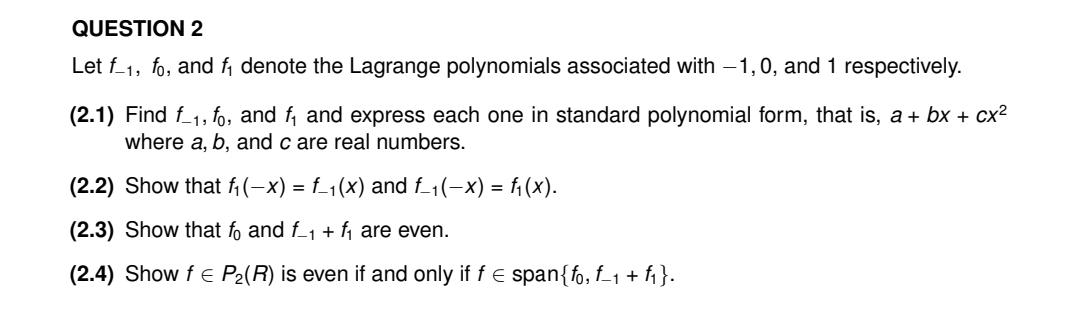# (Solved): Let f1,f0, and f1 denote the Lagrange polynomials associated with 1,0, and 1 respec ...Let , and denote the Lagrange polynomials associated with , and 1 respectively. (2.1) Find , and and express each one in standard polynomial form, that is, where , and are real numbers. (2.2) Show that and . (2.3) Show that and are even. (2.4) Show is even if and only if .

We have an Answer from Expert

We know that ,If the data point are    then
Lagrange polynomial is defined as:

(2.1) :
We have to find    and    and express in the standard form   .
Here the Lagrange polynomial associated with    are

We have an Answer from Expert